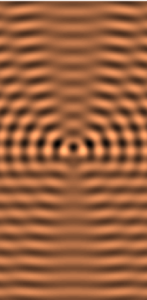# Scattering theory – multipoint formulas

The paper titled “Multipoint formulas for scattered far field in multidimensions” was published in “Inverse Problems” (Q1 WoS) journal. Novikov R.G. Multipoint formulas for scattered far field in multidimensions // Inverse Problems. 2020. V. 36. № 9. Article 095001. DOI:10.1088/1361-6420/aba891

The author gives, in particular, asymptotic formulas for finding the scattering amplitude at fixed frequency and angles (scattered far field) from the scattering wave function given at _n_ points in dimension _d_≥ 2. These formulas are extremely explicit and their precision is proportional to _n_. The results use, in particular, the theory of Vandermonde matrices.